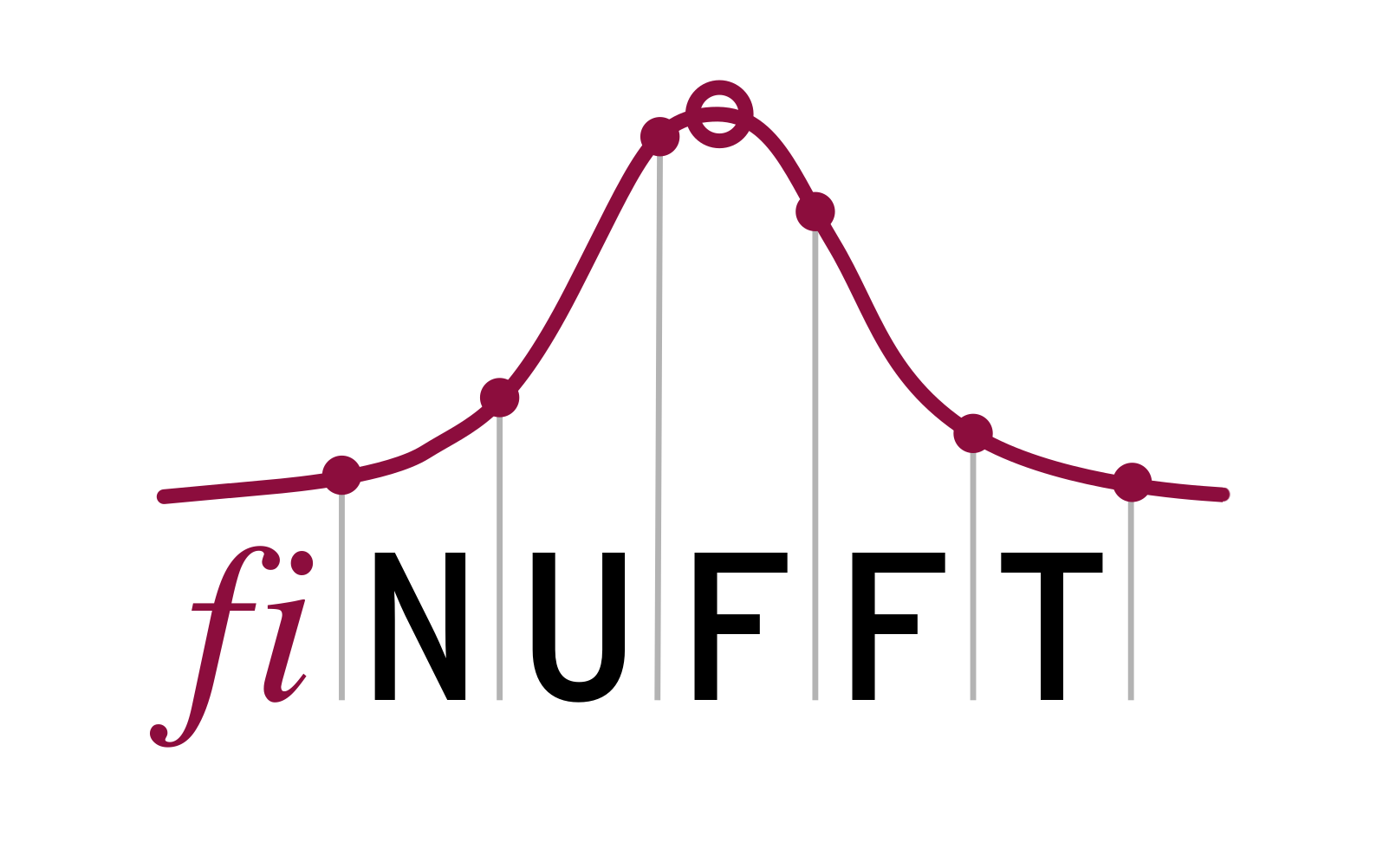# Flatiron Institute Nonuniform Fast Fourier Transform¶FINUFFT is a set of libraries to compute efficiently three types of nonuniform fast Fourier transform (NUFFT) to a specified precision, in one, two, or three dimensions, on a multi-core shared-memory machine. The library has a very simple interface, does not need any precomputation step, is written in C++ (using OpenMP and FFTW), and has wrappers to C, fortran, MATLAB, octave, and python. As an example, given $$M$$ arbitrary real numbers $$x_j$$ and complex numbers $$c_j$$, with $$j=1,\dots,M$$, and a requested integer number of modes $$N$$, the 1D type-1 (aka “adjoint”) transform evaluates the $$N$$ numbers

(1)$f_k = \sum_{j=1}^M c_j e^{ik x_j}~, \qquad \mbox{ for } \; k\in\mathbb{Z}, \quad -N/2 \le k \le N/2-1 ~.$

The $$x_j$$ can be interpreted as nonuniform source locations, $$c_j$$ as source strengths, and $$f_k$$ then as the $$k$$th Fourier series coefficient of the distribution $$f(x) = \sum_{j=1}^M c_j \delta(x-x_j)$$. Such exponential sums are needed in many applications in science and engineering, including signal processing, imaging, diffraction, and numerical partial differential equations. The naive CPU effort to evaluate (1) is $$O(NM)$$. The library approximates (1) to a requested relative precision $$\epsilon$$ with nearly linear effort $$O(M \log (1/\epsilon) + N \log N)$$. Thus the speedup over the naive cost is similar to that achieved by the FFT. This is achieved by spreading onto a regular grid using a carefully chosen kernel, followed by an upsampled FFT, then a division (deconvolution) step. For the 2D and 3D definitions, and other types of transform, see below.

The FINUFFT library achieves its speed via several innovations including:

1. The use of a new spreading kernel that is provably close to optimal, yet faster to evaluate than the Kaiser-Bessel kernel